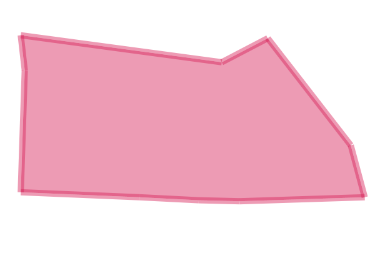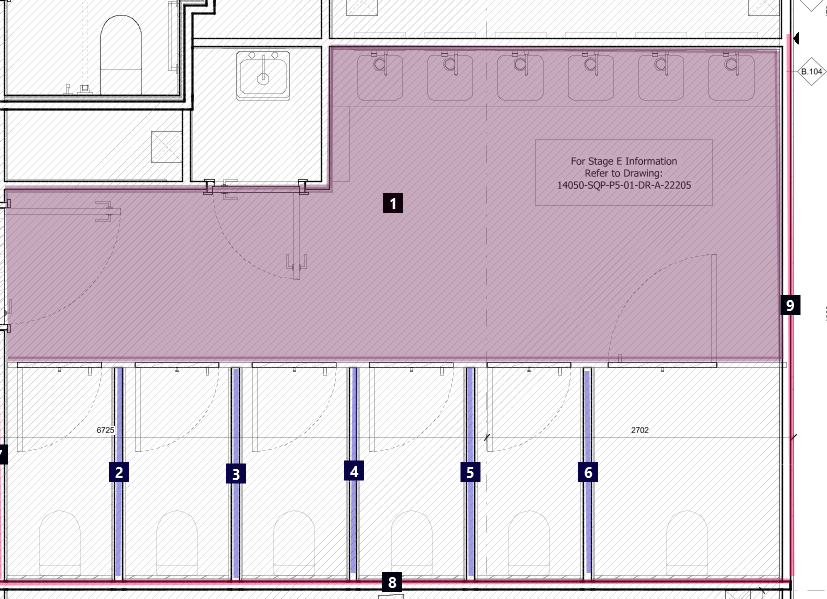# B4J QuestionB4XCanvas Problems

#### keirS

##### Well-Known Member
Longtime User
This code.

B4X:
``````Sub Process_Globals
Private fx As JFX
Private MainForm As Form
Dim CVS As B4XCanvas
Dim RPane As B4XView
Dim Xui As XUI
End Sub

Sub AppStart (Form1 As Form, Args() As String)
MainForm = Form1
MainForm.Show
RPane = MainForm.RootPane
CVS.Initialize(RPane)

Dim Points As List
Points.Initialize

Dim P1 As Point
Dim p2 As Point
Dim P3 As Point
Dim P4 As Point
Dim P5 As Point
Dim P6 As Point
Dim P7 As Point
Dim P8 As Point
Dim P9 As Point
Dim P10 As Point
P1.Initialize(300.6000061035156,149.8000030517578)
p2.Initialize(461.3999938964844,171.39999389648438)
P3.Initialize(498.20001220703125,152.1999969482422)
P4.Initialize(564.5999755859375,237.8000030517578)
P5.Initialize(575.7999877929688,279.3999938964844)
P6.Initialize(475.79998779296875,282.6000061035156)
P7.Initialize(443, 281.79998779296875)
P8.Initialize(395.79998779296875, 279.3999938964844)
P9.Initialize(300.6000061035156, 275.3999938964844)
P10.Initialize(303.79998779296875,177.8000030517578)

DrawPoints(CVS,Points,1943610202,5,True)
DrawFilledPath(CVS,Points,1943610202,5)

Log(ShoelaceArea(Points))

Dim R As B4XRect
R.Initialize(10,-10,20,10)
Log(R.Height)
Log(2 * cPI * 9)
Log(cPI * Power(5,2))

End Sub

'Return true to allow the default exceptions handler to handle the uncaught exception.
Sub Application_Error (Error As Exception, StackTrace As String) As Boolean
Return True
End Sub

Sub DrawPoints(TargetCanvas As B4XCanvas,Pts As List,Color As Int,StrokeWidth As Float,Final As Boolean)
Dim StartPX As Double = -1
Dim StartPY As Double = -1
Dim EndPX As Double = -1
Dim EndPY As Double = -1

Dim Cntr As Int = 0
For Each P As Point In Pts
If StartPX = - 1 Then
StartPX = P.XCoord
StartPY = P.YCoord
Else
EndPX = P.XCoord
EndPY = P.YCoord
End If
Cntr = Cntr + 1

If StartPX > 0 And EndPX > 0 Then
TargetCanvas.DrawLine(StartPX,StartPY,EndPX,EndPY,Color,StrokeWidth)
StartPX = EndPX
StartPY = EndPY

End If

Next
Dim Cntr As Int = 0
If Not(Final) Then
For Each P As Point In Pts
Cntr = Cntr + 1
If StartPX = - 1 Then
StartPX = P.XCoord
StartPY = P.YCoord
TargetCanvas.DrawCircle(StartPX,StartPY,10,Xui.Color_Black,True,StrokeWidth)
TargetCanvas.DrawText(Cntr,StartPX,StartPY + 4,Xui.CreateDefaultBoldFont(16),Xui.Color_White,"CENTER")

Else
EndPX = P.XCoord
EndPY = P.YCoord
TargetCanvas.DrawCircle(EndPX,EndPY,10,Xui.Color_Black,True,StrokeWidth)
TargetCanvas.DrawText(Cntr,EndPX,EndPY + 4,Xui.CreateDefaultBoldFont(16),Xui.Color_White,"CENTER")

End If
Next
End If
End Sub

Sub DrawFilledPath(TargetCanvas As B4XCanvas,Pts As List,Color As Int,StrokeWidth As Float)
Dim Path As B4XPath
Dim P As Point = Pts.Get(0)
Path.Initialize(P.XCoord,P.YCoord)

For I = 1 To Pts.Size - 1
Dim P As Point = Pts.Get(I)
Path.LineTo(P.XCoord,P.YCoord)
Next

CVS.DrawPath(Path,Color,True,StrokeWidth)

End Sub
Sub ShoelaceArea(Pts As List) As Double
Dim ARea As Float = 0
For I = 0 To Pts.Size - 2
Dim Pt1  As Point = Pts.Get(I)
Dim Pt2 As Point = Pts.Get(I+1)

ARea = ARea + (Pt1.XCoord * Pt2.YCoord) - (Pt2.XCoord * Pt1.YCoord)
Next
Dim StartPoint As Point = Pts.Get(0)
Dim Endpoint As Point = Pts.Get(Pts.Size -1)

If StartPoint.XCoord = Endpoint.XCoord And StartPoint.YCoord = Endpoint.YCoord Then
Return Abs(ARea /2)
Else
Return Abs(ARea + (Endpoint.XCoord * StartPoint.YCoord) - StartPoint.XCoord * Endpoint.YCoord) / 2.0

End If
End Sub``````

Produces this shapeThere are a couple of problems with this

1. The lines and fill are using the same color. They are not the same color in the image.
2. The fill is exceeding the boundaries of the edges of the coordinates used.So how can I get the colors to match and get the fill not to exceed the coordinate boundaries?

#### Erel

##### B4X founder
Staff member
Longtime User
Why aren't you using hex colors?
The hex value is: 0x73D9235A

Now you can immediately see that the alpha channel is not FF = it is semitransparent. So every "layer" makes it less transparent. First step is to use an opaque color.

#### keirS

##### Well-Known Member
Longtime User
The code is an example. The colors are picked by the user using B4Xcolor picker. I just copied and pasted the color from a saved file. The user will pretty much always want to use semitransparent colors because they are tracing over construction drawings and need to see the original detail:Even when there is no fill there is more than the passed color being drawn.#### klaus

##### Expert
Longtime User
When you draw lines, parts of them are overlapping in the corners.
Therefore the color gets darker.
Have you tried to draw only the filled polygon without drawing the lines, there is probably no overlapping.

Last edited:
•Erel

#### keirS

##### Well-Known Member
Longtime User
The lines and the fill are two separate things. The lines indicate a linear measure item (walls in this case) the fill indicates an area measure item (a ceiling). As per the example above they are assigned individual numbers. It’s quite complex but in this case the colouring indicates a particular phase of construction is complete.

#### klaus

##### Expert
Longtime User
When you draw the outline you can use DrawPath with Filled = False.
In that case there is no overlapping in the corners.
Be aware that B4XCanvas DrawPath automatically closes the path to a polygon and clips the area.
Which means that, with DrawPath, you cannot draw a polyline and nothing is drawn outsides the polygon.

If your program is only for B4J you might have a look at the jCanvasExt library, which extends the Java Canvas methods not exposed in B4J.

Anyway, with semi-transparent colors, any overlapping will darken the color.

•DonManfred and Erel